I. INTRODUCTION.

PHYSICS, PHYSICISTS AND ENGINEERS

What is physics? It is the science of nature. What is science? Science is what people know about the structure and behavior of our world. About where a rainbow, clouds, wind, lightning come from. How lasers and motors work. And many many others. Therefore, when you ask “Why does the motor work?”, “Where do the northern lights come from?”, “Why does the water have no shape like a piece of ice, but takes the form of a vessel into which it is poured?”, “Where does the electrical current come from?” - you ask questions of physics. Accordingly, the person whose work it is to answer these questions is called a physicist. In addition to physics, there are other sciences. For example, the science of animals, microbes, plants is called biology. People involved in science (physics, biology, geology, etc.) are also called scientists. To answer the questions “why?” and “where?”, scientists explore nature and natural phenomena and thus develop science, expand our scientific knowledge about the world around us.

In addition to scientists, there are engineers. A person whose job is to make certain devices and who knows how to make them is called an engineer. They put scientific knowledge into practice. For the engineer, the main question is "how to do it?" For example, how to make an airplane, how to make a computer, how to build a house, etc. .   Doctors, psychologists, geologists, pharmacists also put into practice scientific knowledges.

The work of a modern scientist and engineer is not possible without a computer and the ability to work with modern tools. This spring, you should make a pneumatic catapult to launch projectiles and investigate the flight of missiles depending on their design. Therefore, in the near future, you will begin to work with mechanical tools and on a computer in order to learn how to make simple cartoons, plot simple graphs, make a rocket with a maximum flight range and understand the principle of caliper operation that you will need in the manufacture of a catapult. Therefore, you will work as physicists and engineers.

The first people who discovered how to build a house, how to make glass and turn it into beads, how to make a sword, bow and arrows, i.e., the first engineers appeared back in Ancient Egypt and Ancient Babylon. Ancient engineers knew how to build giant temples, how to make beautiful jewelry, how to make finger or eye prosthetics, how to make quick chariots or sharp swords (see Fig. 1). They knew how to do it, but the question “why should we do this and not otherwise?” was of little interest.Fig.1. Artifacts of Ancient Egypt and Babylon

The first to become interested with these questions were the ancient Greeks.  In asking the questions of  “Why do you need to do just that?”, “How is the world around us structured?” the ancient Greeks created the foundations of most sciences, and among them was physics. The first physicist to create the very concept of physics was Aristotle. He lived almost 2500 years ago. But of course, real progress in the development of physics and technology came one and a half thousand years after the birth of Christ. So much time was required for the new Christian countries of Europe to grow stronger, and for people to emerge in them with a new culture of relationships.

This video will tell you about the most ancient civilization, Ancient Egypt:

 `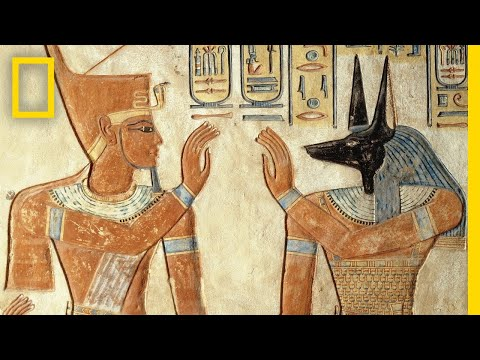` Ancient Egypt

When the first city-states of Ancient Greece appeared, the Egyptian kingdoms already existed for more than 2 thousand years. These ancient Egyptian kingdoms were as ancient for the ancient Greeks as the ancient Greeks are for us. This video will tell you about main  advantages of Ancient Greece:

 `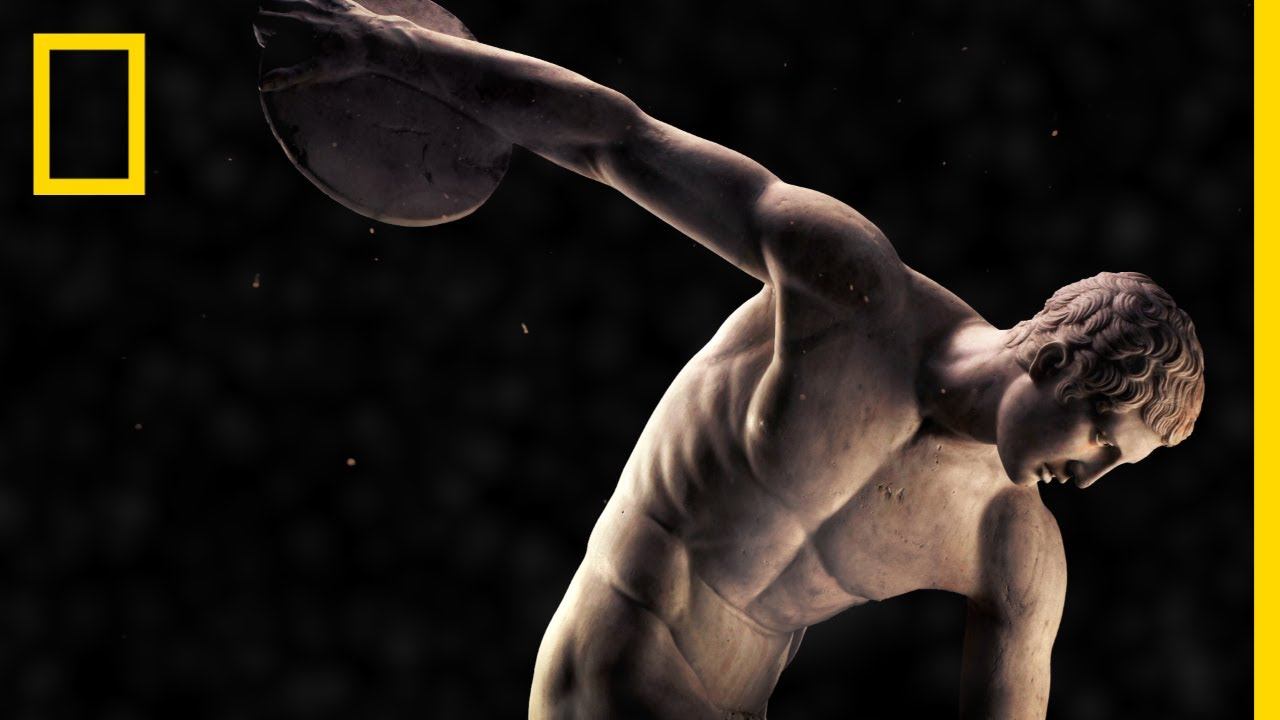` Ancient Greece

The following video will tell you about some of the most outstanding scientific discoveries that define our modern worldview, according to the authors of the video. It is only necessary to make two remarks about this video. Mentioning A. Einstein and his Theory of Relativity, they talked about the Special Theory of Relativity and the constant speed of light postulate. The authors of this theory and postulate are H. Lorentz and H. Poincare.

The second remark is related to Darwin's theory. The video presents Darwin's interpretation of the law he discovered on the effect of natural selection on the diversity of species of living organisms. The law itself is beyond doubt. But it should be noted, that there is another possible interpretation, saying that the possibility of mutability is inherent in the genome of living organisms. According to this interpretation, the process of transforming a fish into a human being, as it can be done according to Darwin's interpretation, is very unlikely. When you learn the simplest programming, we will explore these two interpretations.

 `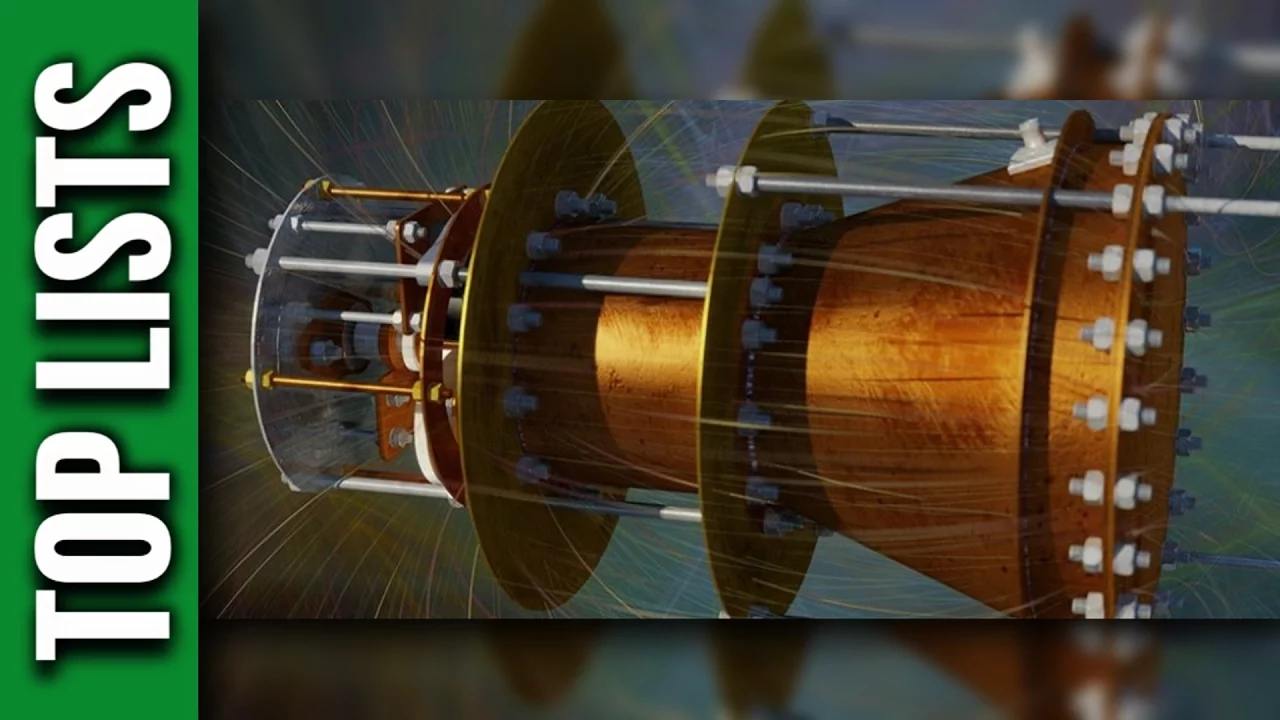` The Most Outstanding Discoveries

This video will tell you about the most interesting engineering developments in the field of robotics.

 `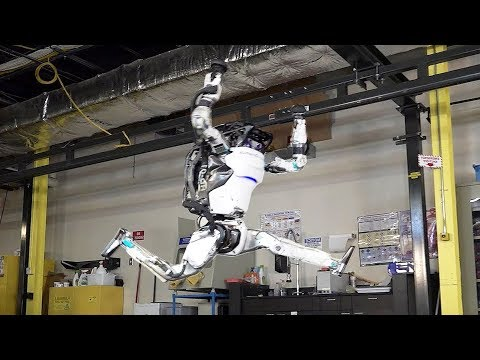` The Most Advanced Robots

And this will tell about the most interesting areas of future engineering developments in medicine:

 `` Top 10 Technologies of Healthcare

PHYSICAL MODELS

So, the physicist seeks to find answers to the questions of how the world works, how this or that device operates, why it is necessary to make a machine this way, and not otherwise. But what does it mean to answer the question “How is it arranged?”, or “How does it work?”, or “why does it happen?”.

For example, suppose we asked three boys the question "why does the incandescent light bulb glow?" The first said that the bulb does not glow, but shines. The light bulb is made of glass. And the glass has a very smooth surface, from which light can be reflected, like from a mirror. Therefore, when the light is reflected, we see that the bulb is shining, and it seems to us that it is glowing.

The second said that the light is on because they pressed the switch.

The third boy said there is a filament, thin and very long wire twisted into a spiral inside the light bulb.  The filament itself consists of very small atoms, around which still many times smaller electrons rotate, and electrons freely running between the atoms along the wire. Electrons orbiting atoms are called electrons bound to an atom. Usually the bulb filament is made of tungsten. For every atom of tungsten, there are 72 bound electrons and 2 free ones. Atoms with bound electrons rotating around them are much heavier much bigger than free-running electrons. They occupied all the space inside the filament, so, they are tightly packed. Therefore, atoms can not move like very small free electrons. They can only oscillate near their place in the filament. When we press the switch, the free electrons in the filament begin to run from one end of the filament to the other. Such a movement of electrons in one direction is called an electronic current. Running they collide with bounded electrons and atoms. From collisions, atoms and bounded electrons begin to oscillate even faster around their place. As a result, the filament heats up.  Due to oscillations of bound electrons and atoms, electromagnetic waves are emitted. When the oscillation frequency of bound electrons and atoms becomes very large, they emit light, which we can see. Our mobile phone also emits electromagnetic waves when electrons oscillate in its antenna. Only the frequency of these oscillations is much smaller and we do not see them.

As you can see, each of the three boys gave his own explanation, or in other words they suggested their own model of the phenomenon. How can we determine which of these explanations are correct? Which one is more scientific? Obviously, the first explanation is wrong. After all, a light bulb can also glow in the dark, when there are no other light sources and there is nothing to reflect. About this explanation, we can say that it is not correct, because it is not logical. Not logical means reasoning, which contradicts common sense, or which is not entirely honest, and we do not believe it.

The second explanation seems more logical. Indeed, the light starts to glow only when the switch is on. But it does not explain where the light came from. After all, if the light bulb burns out, then the same flipping of a switch will do nothing. Therefore, by logical reasoning, it turns out that it is also not correct.

The third explanation seems logically correct. But we are not able to test this logic, because we do not know why the oscillating electrons emit light. We do not know what atoms and electrons are. While we can accept this explanation, it can only be done on faith.

This video will illustrate the most correct, physical explanation of the third boy and tell how incandescent bulbs are made:

 `` Light Bulb Production

And this will show how the engineer would answer what a bulb is and how to make it:

 `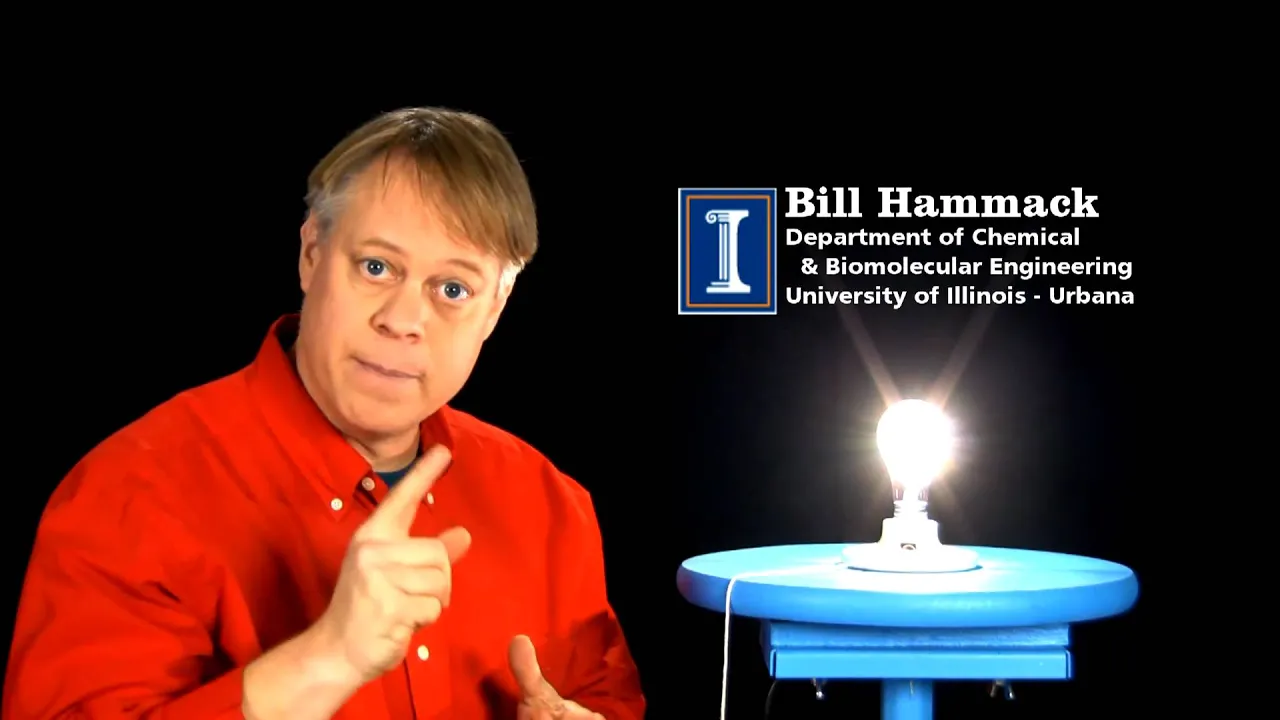` Light Bulb Filament

PHYSICS, MATHEMATICS AND LOGIC

What conclusion can we draw from the given story? For a scientific explanation, logic is needed in reasoning. The field of human knowledge dealing with logic and logical reasoning is called mathematics. Without mathematics, no modern science is possible. Mathematics has developed its own language to carry out logical reasoning. There are no ordinary objects that we see around: bags, cars or people. There are no ordinary actions that are performed on these objects in ordinary life, such as bring, go, push. Mathematics has its own mathematical objects: numbers, mathematical points, number lines, planes, spaces, sets, domains etc.. Actions on these mathematical objects are called logical or mathematical operations. You are already familiar with some of these operations. For example, this is addition, subtraction, multiplication, division, equality. In the language of mathematical objects and mathematical operations, you can tell any of our history. And with the help of mathematics, you can not only talk about the phenomena of physics, but also calculate how much material you need to take, or what force you need to apply to get the desired result. For example, in the language of mathematics, the whole story about a light bulb with a filament in vacuum emitting light can be written as follows:

P=I*U

T=(P-Pi)/(c*m)

Pi=А*σ*ε*(T4- T04)

λ=b/T

Moreover, this short record will not only tell how the bulb works, but also allows you to calculate the temperature of the thread. Knowing the temperature, we can determine whether we will see its light radiation or it will be infrared radiation, which our eyes do not distinguish. And if we use the Planck law, we can determine how brightly it will shine for us. In addition, the mathematical record will tell you how best to make a light bulb, and what materials are best suited for the wire. But for now, these four written equations do not tell us anything. We have yet to learn to speak the language of mathematics.

Now you also know what a model of a phenomenon in physics is. In our story, each of the boys presented his own model of the phenomenon of the glow of a light bulb thread. So, the model of the phenomenon is an explanation of the phenomenon. When the phenomenon is very important for most scientists, for example, the phenomenon of body attraction by the Earth, the model of the phenomenon is called a theory. The main stimulus for the development of science is finding logic in natural phenomena. Therefore, modern scientific models must be presented in the language of mathematics. Only in this case we can honestly say that we logically and correctly explained the phenomenon.

But not all phenomena lend themeselves to this interpretation. Therefore, there are simplest models of phenomena that contain only a description and systematization of phenomena. For example, science still does not understand how living organisms were created. Therefore, the simplest model is used, which only describes animals and plants, and breaks them into classes, i.e. systematizes them. For example, there are classes of mammals, reptiles, amphibians and others. In turn, classes are divided into orders. The class of mammals is divided into orders of artiodactyls, carnivores, rodents, primates and others. The development of science is associated not only with the discoveries of new phenomena, but also with the development of models. After all, if there were no models, then we would never be able to distinguish new phenomena from old ones.

This can be illustrated using the library as an example. Everyone can come there. Therefore, before giving out the books, the librarian needs to know whether this is a new person or he is already registered in the library. In this case, a record of a person in the database is the simplest model of a person. The model explains to us who this man is, what his name is, what his age is, where he lives, what books he took earlier. Without this model, the librarian will not be able to say whether this is a new visitor or not.

In the development of physical models and science, paradoxes played an important role. A paradox is a statement or phenomenon from which a conclusion logically follows that contradicts our original understanding. Therefore, paradoxes force us to modify our models of phenomena. Without paradoxes, there would be no development of science.

1. The paradox of dichotomy or the paradox of dividing into two.The paradox led humanity to the understanding that between any two points of a line there should always be an infinite number of other points. This became the basis for understanding the properties of continuity in mathematics and physics.
2. Achilles and the tortoise. The paradox led to the understanding that the continuous movement of the body means movement in any period of time, no matter how small this period of time is.
3. The flying arrow. The paradox explores how the speed of the body changes over any infinitely small period of time. In other words, Zeno again trying to figure out what continuous movement is. The paradox led to the emergence of such scientific concepts as speed, derivative, tangent.
4. The moving rows or a stadium. The paradox has led to the understanding that movement is relative. Without this paradox, there would be neither the Galilean relativity principle, nor Newtonian mechanics, nor the Special Theory of Relativity.
5. The paradox of the place. The paradox influenced our creation of theories of infinite number series and sets of elements, the creation of the concept of sums of infinite series, and the calculation of the areas of different figures.
6. The paradox of millet grain (another name is the paradox of the pluralities). He also influenced our understanding of the sum of infinite number series and the development of computer computational methods.

Zeno's paradoxes can be seen here:

 `` Zeno's paradoxes

Zeno's paradoxes show that man cannot imagine either an infinitely large value or an infinitesimally small one. We cannot imagine them, because in our everyday life everything is finite. Even the distance to the farthest star and the size of the atomic nuclear particles, quarks, are finite. Nevertheless, man has been given this understanding, although this is not around him. And he not only understands what is infinitely small or infinitely large, but also uses these concepts in his life.

When you are riding in a car, its speed is determined using Hall sensors. These sensors would not exist if people did not know about infinitely large and infinitesimal quantities. Many engineering calculations use the number π. Its value is equal to half the length of a circle with a radius of 1. Despite this clarity, the number π can only be accurately calculated using the sum of an infinite number series. Therefore, we will never know its exact value, and should use its approximate value. From the prospective of mathematics, we are dealing with infinite quantities when the centrifuge of the dryer dries the laundry, or when the rocket flies into space.

Thus, another paradoxical contradiction arises. On the one hand, there are objects whose existence can be proved logically. On the other hand, we cannot imagine, measure, feel them, because no one has ever seen them, but we can use them.

This is a global paradox of our worldview, which leads to the question of how real our logic and our mathematical models of physical phenomena are. Even our example has shown that different models may arise to describe the same phenomenon. Some of them can be checked by logical reasoning. But for others, for example, as in the case of the third boy, we will need measurements and additional observations to verify the proposed explanation.

Any measurements and observations in science are called experiments. Only through experiments can we determine whether it is true that electrons and atoms exist,  that an electromagnetic wave is emitted during the oscillation of electric charges, that light is also an electromagnetic wave. An experimental verification will also be required for the legitimacy of using each of the written equations of this model. Therefore, all scientific models and theories must be verified experimentally.

But with mathematics, the situation is different. Mathematical theories and proofs do not require experimental verification. They are proved only logically. And if our experiment showed that the proposed equations lead to incorrect results, then we would not change the equations, we would change our entire physical model. And the new model would use different equations already. Since mathematics does not require verification by experiment, mathematics is actually not a science.

“It turns out that our logic exists regardless of experiment. But how then is mathematics used to describe models of reality and the laws of physics? It turns out the same paradox of our worldview, ”you say. Yes, and humanity has not yet come to a unified solution to this paradox. Some people believe that logic exists only in our head, but does not exist in reality. Therefore, the laws of physics also exist only in our head. These laws are the same for everyone because there is a collective mind. Therefore, one should not be surprised at the discrepancy between mathematical conclusions and reality. Of course, then we need to explain why we need non-existing laws, and how then, for example, any body on Earth knows that it should be attracted to the Earth by a certain rule. This explanation is very long and not simple. Its consideration would take more than one year. We will not consider it here.

The other part, believes that there is logic in the phenomena of our world, physical laws really exist, and the scientist’s job is to search for logic in natural phenomena. Therefore, the use of mathematics in science is justified. And since logic really exists in our world, then mathematical logic is the same for everyone. We can say that logic is another property of our world that we can know. This property does not depend on the fallacies of an individual person, and measurements are not required to test mathematical theories and logical operations, but our mind is required. Therefore, we can study the properties of continuous, infinitesimal and infinitely large quantities and apply them. And despite the fact that we cannot touch them with the help of our 5 senses, we can imagine them mentally.

Which group of people you belong to is your personal decision.

NUMBER LINE

Understanding Zeno's paradoxes gave us such important mathematical concepts as a numerical line, a mathematical point, a continuous line, speed, and the area of arbitrary figures.These mathematical concepts are successfully used in modern physics to describe the properties of objects and phenomena surrounding us.

The following video tells what a number line is: https://youtu.be/9OzENFeK8GI

On this number line, you can find not only integers, but any other numbers. For example, consider fractional numbers.

What is a fraction number? Suppose you are given a fractional number 3/4. It means that we took the integer 1. Say we took one cake. Then we cut it into 4 equal parts and took 3 slices. These 3 slices will be ¾ from the whole cake. Similarly, 2/7  will mean that we cut the cake into 7 parts and took 2 slices. The number 5 ¾ consists of the number 5 plus the fractional number ¾. It means that we took 5 whole cakes and added 3 slices to it from the sixth cake, which was cut into 4 parts.

The following video shows how fractional numbers are laid out on a number line: https://youtu.be/fLY9yRdBObQ

Accordingly, the fractional number 5 ¾ will be the red dot shown on the number line (Fig.2):Fig.2. Number Line

` `